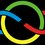# International Mathematical Olympiad '61, Second Day

The goal of this set of notes is to improve our problem solving and proof writing skills. You are encouraged to submit a solution to any of these problems, and join in the discussion in #imo-discussion on Saturday 14 at 9:00 pm IST ,8 30 PDT. For more details, see IMO Problems Discussion Group.

Here is the next note in the collection, A whole lot of interesting Olympiad Geometry! Post any progress you make and Enjoy!

4. (GDR) In the interior of $\Delta P_1P_2P_3$ a point $P$ is given. Let $Q_1, Q_2,$ and $Q_3$ respectively be the intersections of $PP_1, PP_2,$ and $PP_3$ with the opposing edges of $\Delta P_1P_2P_3$. Prove that among the ratios $PP_1 / PQ_1, PP_2/PQ_2,$ and $PP_3/PQ_3$ there exists at least one not larger than 2 and at least one not smaller than 2.

5. (CZS) Construct a triangle $ABC$ if the following elements are given: $AC = b, AB = c,$ and $AMB = \omega (\omega < 90^{\circ})$, where $M$ is the midpoint of $BC$. Prove that the construction has a solution if and only if $b \tan{\dfrac{\omega}{2}} \leq c < b$. In what case does equality hold?

6. (ROM) A plane $\epsilon$ is given and on one side of the plane three noncollinear points $A, B,$ and $C$ such that the plane determined by them is not parallel to $\epsilon$. Three arbitrary points A', B', and $C'$ in $\epsilon$ are selected. Let $L, M,$ and $N$ be the midpoints of AA', BB', and $CC'$, and $G$ the centroid of $\Delta LMN$. Find the locus of all points obtained for G as A', B', and $C'$ are varied (independently of each other) across $\epsilon$

###### This is part of the set International Mathematical OlympiadsNote by Sualeh Asif
4 years, 3 months ago

This discussion board is a place to discuss our Daily Challenges and the math and science related to those challenges. Explanations are more than just a solution — they should explain the steps and thinking strategies that you used to obtain the solution. Comments should further the discussion of math and science.

When posting on Brilliant:

• Use the emojis to react to an explanation, whether you're congratulating a job well done , or just really confused .
• Ask specific questions about the challenge or the steps in somebody's explanation. Well-posed questions can add a lot to the discussion, but posting "I don't understand!" doesn't help anyone.
• Try to contribute something new to the discussion, whether it is an extension, generalization or other idea related to the challenge.

MarkdownAppears as
*italics* or _italics_ italics
**bold** or __bold__ bold
- bulleted- list
• bulleted
• list
1. numbered2. list
1. numbered
2. list
Note: you must add a full line of space before and after lists for them to show up correctly
paragraph 1paragraph 2

paragraph 1

paragraph 2

[example link](https://brilliant.org)example link
> This is a quote
This is a quote
    # I indented these lines
# 4 spaces, and now they show
# up as a code block.

print "hello world"
# I indented these lines
# 4 spaces, and now they show
# up as a code block.

print "hello world"
MathAppears as
Remember to wrap math in $$ ... $$ or $ ... $ to ensure proper formatting.
2 \times 3 $2 \times 3$
2^{34} $2^{34}$
a_{i-1} $a_{i-1}$
\frac{2}{3} $\frac{2}{3}$
\sqrt{2} $\sqrt{2}$
\sum_{i=1}^3 $\sum_{i=1}^3$
\sin \theta $\sin \theta$
\boxed{123} $\boxed{123}$

Sort by:

@Sharky Kesa @Xuming Liang Geometry this time!

- 4 years, 3 months ago